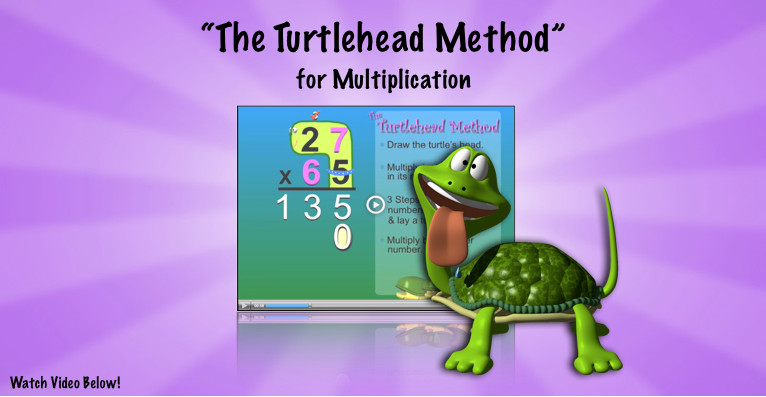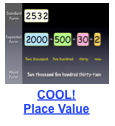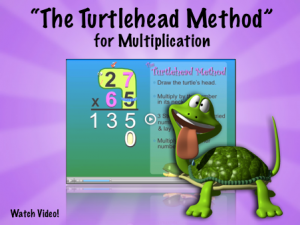##### Print or Share Flyer## 25 Jul Multiplication Video- “The Turtlehead Method”### Memorable method for multi-digit Multiplication!

This Multiplication video introduces students to the “Turtlehead Method”.  The Turtlehead Method provides a simple and entertaining way for students to recall the steps involved in solving multi-digit Multiplication problems. featured Factivation!® Teacher Tube Multiplication video also illustrates the importance of having mastered single-digit Multiplication facts, as students learn how to apply the basic facts to larger Multiplication problems.

## Using the Turtlehead Multiplication video in the classroom

Teachers/parents are invited to use this Multiplication video as a fun introduction to multi-digit Multiplication, leading into a follow-up class discussion of WHY the “turtle egg” (the Zero) is needed:  Guide students in reflecting on previous learning involving place value.  Pause the video on one of the multi-digit problems and ask students to identify the true value of each digit represented in the problem.   Ask: If the bottom number has a “3” in the tens place, what are we actually multiplying by?  (30)Need to review place value?  Our COOL! Place Value Video also received a 5-apple Teacher Tube rating.  Use this video to teach or review place value to the thousands.

You’ll find that students will ask to watch the Turtlehead Multiplication video often. Multiple viewings followed by paper/pencil or iPad practice sessions are encouraged, as each provides review of the computational steps needed to solve multi-digit Multiplication problems.

CCSS.Math.Content.4.NBT.B.5 Multiply a whole number of up to four digits by a one-digit whole number, and multiply two two-digit numbers, using strategies based on place value and the properties of operations. Illustrate and explain the calculation by using equations, rectangular arrays, and/or area models.

##Want More Multiplication Videos?

We have 28 more Multiplication videos plus 9 accompanying Division videos!  Check out our free Multiplication/Division lessons and start getting your students fully factivated today!  Lessons include instructional videos, CCSS-aligned printables, Smartboard games, and assessments– both paper/pencil and online!